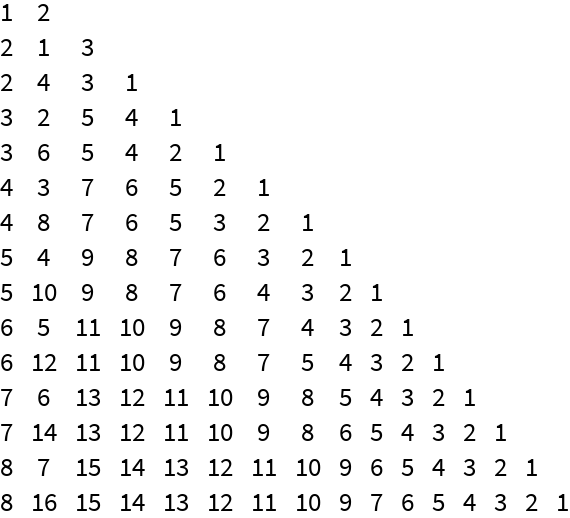#Function Repository Resource:

# PermutationFromIndex

Give the permutation corresponding to a given length and lexicographic index

Contributed by: Ed Pegg Jr
 ResourceFunction["PermutationFromIndex"][index,length] gives the permutation with a given lexicographic index and length.

## Details and Options

Permutations are considered to be in normal sorted order.
Lehmer codes are generated as an intermediary step.
The generating algorithm for Lehmer codes is as follows. For each number in the permutation, count how many subsequent values it exceeds.

## Examples

### Basic Examples

Give the first permutation of length 4:

 In:=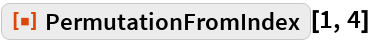Out=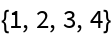Give the last permutation of length 4:

 In:=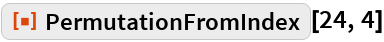Out=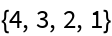Give the 777th permutation of length 7:

 In:=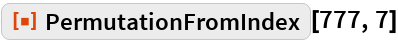Out=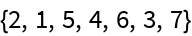### Scope

Permutations with an arbitrarily large lexicographic index can returned:

 In:=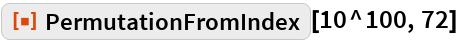Out=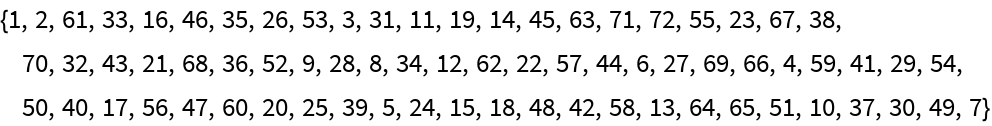### Possible Issues

When an index for length n exceeds n! the index is considered (mod n!):

 In:=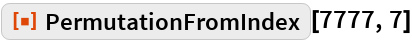Out=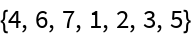In:=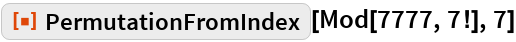Out=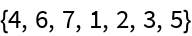### Neat Examples

Permutations halfway through the index:

 In:=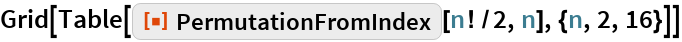Out=